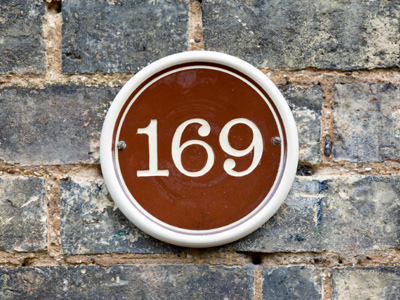13 × 13 = 169.

# Properties of Numbers 2

This Math quiz is called 'Properties of Numbers 2' and it has been written by teachers to help you if you are studying the subject at elementary school. Playing educational quizzes is an enjoyable way to learn if you are in the 3rd, 4th or 5th grade - aged 8 to 11.

It costs only \$12.50 per month to play this quiz and over 3,500 others that help you with your school work. You can subscribe on the page at Join Us

Some numbers are prime numbers - some numbers have other properties.As you continue through school and math lessons, you'll learn a lot more about numbers, and if you choose to go further with math when you leave school, no doubt you will come across some strange terms for numbers. Most likely, you've heard of infinity ~ this means without end ~ and numbers go on forever. Saying this, you will learn about Graham's number, TREE(3) and a googolplex!

For now, we are dealing with the easy stuff, so get 10 out of 10 in this quiz!

1.
Which of the following statements is wrong?
Two is an even number and a prime number
Other than the number 2, there are no even prime numbers
If you add two odd numbers, you get another odd number
If you add two even numbers, you get another even number
7 + 5 = 12 which is not odd. If you want to show that a statement is not always true, find one example that shows it is wrong - like we did here
2.
For those of you with stamina: How many prime numbers are there between 1 and 100 inclusive?
29
26
27
25
Well done those of you who bothered to do this question. The rest of you - do it later. There are 25 prime numbers between 1 and 100. Here they are: 2, 3, 5, 7, 11, 13, 17, 19, 23, 29, 31, 37, 41, 43, 47, 53, 59, 61, 67, 71, 73, 79, 83, 89 and 97. Phew! Does anyone want to find out how many prime numbers there are between 1 and 1,000 inclusive? Just joking! Here's the answer anyway: 168 prime numbers. I wonder if your teacher knows that? By the way, prime numbers are a big thing in higher math
3.
Which of the following statements is wrong?
If a number is divisible by 9, then it is divisible by 3
If you add two prime numbers together, you get another prime number
If you subtract 1 from an even number, you get an odd number
There are four prime numbers between 1 and 10
11 + 7 = 18 which is not a prime number. If you want to show that a statement is not always true, find one example that shows it is wrong - like we did here
4.
Which two prime numbers have to be added together to give 38?
11 + 27
19 + 19
23 + 15
29 + 9
This is the only choice that has two prime numbers
5.
Which of the following statements is wrong?
13 is an odd number
13 × 13 = 159
13 is a prime number
13 is the sixth smallest prime number
13 × 13 = 169! D'oh! By the way, just because 13 is odd, this doesn't prevent it from being a prime number too
6.
Which number is the odd man out: 49, 15, 41, 9?
9
15
49
41
They are all odd, but 41 is also a prime number: a prime number is a positive whole number that is only divisible by 1 and itself but does not include the number 1
7.
What is the smallest prime number?
2
1
0
-1
A prime number is a positive whole number that is only divisible by 1 and itself but does not include the number 1, e.g. 2, 3, 17, 41
8.
Which number is the odd man out: 64, 32, 144, 100?
144
64
100
32
They are all even numbers, but 32 is not a square number. A square number (perfect square) is a number formed by the multiplication of another number with itself, e.g. 144 = 12 × 12; 64 = 8 × 8; 100 = 10 × 10. Note: 1 × 1 = 1
9.
Which number is the odd man out: 71, 17, 11, 39?
11
17
39
71
They are all odd numbers, but only 39 is a non prime number: a prime number is a positive whole number that is only divisible by 1 and itself but does not include the number 1
10.
How many prime numbers are there between 1 and 30?
10
12
11
9
Here they are: 2, 3, 5, 7, 11, 13, 17, 19, 23, 29
Author:  Frank Evans